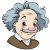## Math Q&A - Novatikaby Teacher

### What is multiplication? Multiplication as repeated addition

How much is 4 times 4? Let’s take 4 multiplied by 4. The first number is the number of donuts in each group. We have four donuts in each group, and the second number is the number of groups. We have four groups of four donuts. that’s four plus four plus four plus four which makes sixteen donuts. So four times four is 16. What is multiplying? Multiplication is something you do with numbers like addition or subtraction. Multiplication is just repeating addition and nothing more. It’s represented by the × simple. Let’s say we want to multiply 3 by